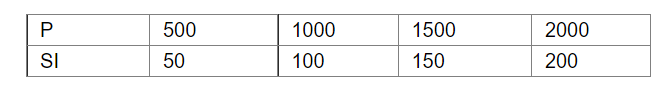# A bank gives 10% simple interest on deposits by senior citizens

Question:

A bank gives 10% simple interest on deposits by senior citizens. Draw a line graph to illustrate the relation between the sum deposited and the simple interest earned.

Find from the graph:

(i) The annual interest obtainable for a investment of Rs 250

(ii) The investment one has to make in order to get an annual simple interest of Rs 70

Solution:

Using $S I=\frac{P \times R \times T}{100}$

Where SI = simple interest

$\mathrm{P}=$ principal

$\mathrm{R}=$ rate of interest

$\mathrm{T}=$ time

We have:(i) Given sum deposited i.e P = 250 , R = 10%, Time = 1 year

using formula we have,

$S I=\frac{250 \times 10 \times 1}{100}$

$S I=25 \mathrm{Rs}$

Hence, the annual interest for an investment of 250 rs is 25 rs.

(ii) Given annual simple interest = 70 rs , R = 10%, T=1 year

using formula we have,

$70=\frac{P \times 10 \times 1}{100}$

$700=P$

Hence, he has to invest $700 \mathrm{rs}$ in order to get an annual simple interest of $70 \mathrm{rs}$